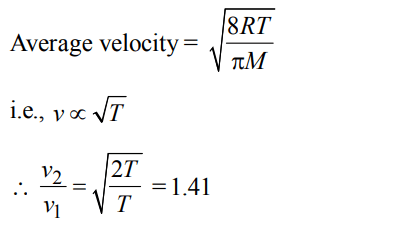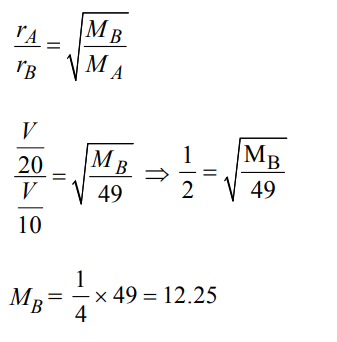## States of Matter Questions and Answers Part-7

1. With rise in temperature, viscosity of a liquid
a) increase
b) decrease
c) remains constant
d) may increase or decrease

Explanation: Visocity $\propto$ 1/temperature

2. With the increasing molecular weight of a liquid, the viscosity
a) decreases
b) increase
c) remains constant
d) All are wrong

Explanation: Visocity $\propto$ molecular weight

3. A weather ballon filled with hydrogen at 1 atm and 27°C has volume equal to 12000 litres. On ascending it reaches a place where the temperature is –23°C and pressure is 0.5 atm. The volume of the balloon is
a) 24000 litres
b) 20000 litres
c) 10000 litres
d) 12000 litres

Explanation:4. If a gas expands at constant temperature, it indicates that :
a) kinetic energy of molecules decreases
b) pressure of the gas increases
c) kinetic energy of molecules remains the same
d) number of the molecules of gas increases

Explanation: At any constant temperature the K.E. of gaseous molecules remains same (K.E. $\propto$ T).

5. The pressure exerted by 6.0g of methane gas in a  $0.03m^{3}$ vessel at 129°C is (Atomic masses : C = 12.01, H = 1.01 and R = 8.314 kpa $dm^{3}k^{-1}mol^{-1}$  )
a) 31684 Pa
b) 215216 Pa
c) 13409 Pa
d) 41777 Pa

Explanation:6. By what factor does the average velocity of a gaseous molecule increase when the temperature (in Kelvin) is doubled ?
a) 2.0
b) 2.8
c) 4.0
d) 1.4

Explanation:7. Two gases A and B having the same volume diffuse through a porous partition in 20 and 10 seconds respectively. The molecular mass of A is 49 u. Molecular mass of B will be :
a) 50.00 u
b) 12.25 u
c) 6.50 u
d) 25.00 u

Explanation:8. A gaseous mixture was prepared by taking equal mole of CO and $N_{2}$. If the total pressure of the mixture was found 1 atmosphere, the partial pressure of the nitrogen $\left(N_{2}\right)$ in the mixture is :
a) 0.5 atm
b) 0.8 atm
c) 0.9 atm
d) 1 atm

Explanation:9. A bubble of air is underwater at temperature 15°C and the pressure 1.5 bar. If the bubble rises to the surface where the temperature is 25°C and the pressure is 1.0 bar, what will happen to the volume of the bubble ?
a) Volume will become greater by a factor of 1.6.
b) Volume will become greater by a factor of 1.1.
c) Volume will become smaller by a factor of 0.70.
d) Volume will become greater by a factor of 2.5

Explanation:10. 50 mL of each gas A and of gas B takes 150 and 200 seconds respectively for effusing through a pin hole under the similar condition. If molecular mass of gas B is 36, the molecular mass of gas A will be :
a) 96
b) 128
c) 20.25
d) 64